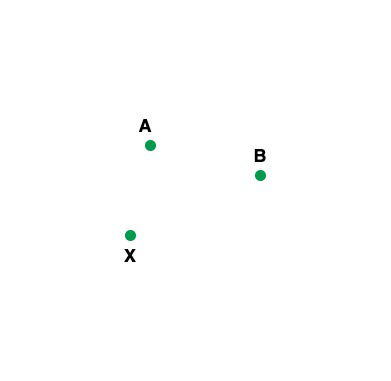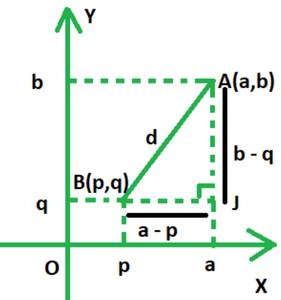# Distance Between Two Points

• Last Updated : 28 Feb, 2022

Coordinate geometry is a sub-branch of mathematics that discusses the relationship between geometry and algebra using graphs comprising curves and lines. Geometric aspects are provided in Algebra, allowing students to solve geometric problems. In coordinate geometry, the coordinates of points on a plane are expressed as an ordered pair of numbers and we can also perform operations like finding the distance between two points, dividing lines in m:n ratios, identifying the mid-point of a line, calculating the area of a triangle in the cartesian plane, and so on. In this article, we will learn how to find the distance between two points.

Points

In mathematics, a location in any space or a plane is known as a point with no size, no height, no length, or not even shape. It is generally used to mark the beginning of a shape or a diagram. Point is represented like as shown in the below image:And the straight path that connects these points are known as lines. The types of points are:

• Collinear points: When 3 or more points are present on the straight line then such types of points as known as Collinear points.
• Non-Collinear points: When a group of points does not present on the same line then such types of points are known as non-collinear points.
• Coplanar Points: When the group of points is present on the same plane then such types of points are known as coplanar points.
• Non-Coplanar Points: When the group of points does not present on the same plane then such types of points are known as non-coplanar points.

### Distance Between Two Points

In general, a distance is used to find how far two living things, non-living things, objects are separated. Similarly in mathematics, distance is used to find the distance between two points according to their given coordinates. It should be noted that the points in question do not necessarily have to be in the same quadrant. The distance formula is generally used in the coordinate system in mathematics to calculate how far two points are present in a coordinate plane which makes it a very important topic in coordinate geometry.

For the sake of simplicity, assume there are two points, A and B, in a coordinate plane, first quadrant. (a, b) are the coordinates of point A, and (a, b) are the coordinates of point B. (p, q). The distance between points A and B abbreviated AB, must be calculated as follows:

AB =Derivation:

Let us assume that two points are present on 2-dimensional plane that is A and B with coordinates (a, b) and (p, q). Now we construct a right angle triangle i.e.AJB in which AB is a hypotenuse. Now we find the distance between point A and B.By Pythagoras Theorem,

AB2 = AJ2 + BJ2

= (a – p)2 + (b – q)2

AB =Example:

Given two points X(5, 10) and Y(2, 4)

So the distance between them is

D =D =D =D =D =So the distance between X and Y is 3√5 units

### Sample Problems

Question 1. Find the distance between points A (4, 6) and B(1, 0).

Solution:

Given: A(4, 6) and B(1, 0).

Now we find the distance between the given points that is A and B

So we use the formula

D =Now put the value in the formula

⇒ AB =units

= 3√5 units

Question 2. Find the distance between points P(4, 0) and Q(1, 0).

Solution:

Given: P(4, 0) and Q(1, 0).

Now we find the distance between the given points that is P and Q

So we use the formula

D =Now put the value in the formula

⇒ PQ =units

= 3 units

Question 3. Given points A(3, 0) and B(1, 0). Find the distance between them.

Solution:

Given: A(3, 0) and B(1, 0).

Now we find the distance between the given points that is A and B

So we use the formula

D =Now put the value in the formula

⇒ AB =units

= 2 units

Question 4. Given points P(6, 0) and R(4, 0). Find the distance between them.

Solution:

Given: P(6, 0) and R(4, 0).

Now we find the distance between the given points that is P and R

So we use the formula

D =Now put the value in the formula

⇒ PR == 2 units

Question 5. Find the distance between the points (12, 0) and (4, 0).

Solution:

Given: P(12, 0) and R(4, 0).

Now we find the distance between the given points that is P and R

So we use the formula

D =Now put the value in the formula

⇒ PR == 8 units

Question 6. Find the distance between the points (12, 0) and (10, 0).

Solution:

Given: A(12, 0) and B(10, 0).

Now we find the distance between the given points that is A and B

So we use the formula

D =Now put the value in the formula

⇒ AB == 2 units

My Personal Notes arrow_drop_up